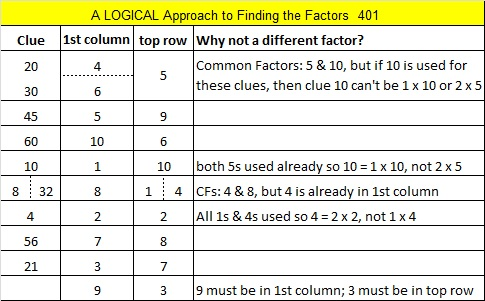# 401 and Level 6

We can easily see that 401 is not divisible by 2, 3, or 5. Is it a prime number? Let’s do a quick test: 2^401 (mod 401) = 2, so 401 is VERY LIKELY a prime number. Scroll down past the puzzle to know for sure.Print the puzzles or type the factors on this excel file: 10 Factors 2015-02-16

• 401 is a prime number.
• Prime factorization: 401 is prime.
• The exponent of prime number 401 is 1. Adding 1 to that exponent we get (1 + 1) = 2. Therefore 401 has exactly 2 factors.
• Factors of 401: 1, 401
• Factor pairs: 401 = 1 x 401
• 401 has no square factors that allow its square root to be simplified. √401 ≈ 20.025How do we know that 401 is a prime number? If 401 were not a prime number, then it would be divisible by at least one prime number less than or equal to √401 ≈ 20.025. Since 401 cannot be divided evenly by 2, 3, 5, 7, 11, 13, 17, or 19, we know that 401 is a prime number.

Pythagorean triples: Since 401 is a prime number, it can only be a part of primitive Pythagorean triples: 40-399-401 and 401-80400-80401.